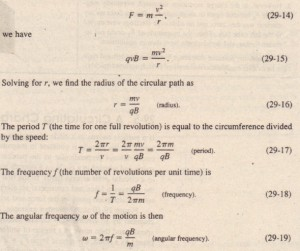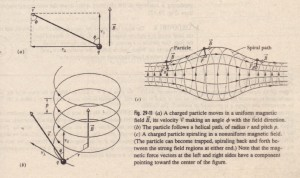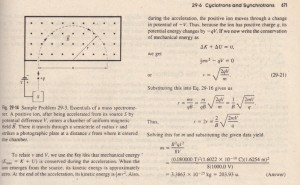# A Circulating Charged Particle

A Circulating Charged Particle

If a particle moves in a circle at constant speed, we can be sure that the net force acting on the particle is constant in magnitude and points toward the center of the circle, always perpendicular to the particle’s velocity. Think of a stone tied to a string and whirled in a circle on a smooth horizontal surface, or of a satellite moving in a circular orbit around Earth. In the first case, the tension in the string provides the necessary force and centripetal acceleration. In the second case, Earth’s gravitational attraction provides the force and acceleration. Figure 29-10 shows another example: A beam of electrons is projected into a  chamber by an electron gun G.The electrons enter in the plane of the page with speed v and then move in a region of uniform magnetic field if directed out of that plane. As a result, a magnetic force FB = qv X if continually deflects the electrons, and because v and if are always perpendicular to each other, this deflection causes
the electrons to follow a circular path. The path is visible in’ the photo because atoms of gas in the chamber emit light when some of the circulating electrons collide with them.

We would like to determine the parameters that characterize the circular motion of these electrons, or of any particle of charge magnitude q and mass m moving perpendicular to a uniform magnetic field JD at speed v. From Eq. 29-3, the force acting on the particle has a magnitude of qvB. From Newton’s second law (1= mG) applied to uniform circular motion (Eq. 6-18),The quantities T,f, and w do not depend on the speed of the particle (provided that speed is much less than the speed of light). Fast particles move in large circles and slow ones in small circles, but all particles with the same charge-to-mass ratio qlm.

take the same time T (the period) to complete one round trip. Using Eq. 29-2, you can show that if you are looking in the direction of 8, the direction of rotation for a positive particle is always counterclockwise, and the direction for a negative particle is always clockwise.

VII = V cos cP and v.l = V sin cPo

The parallel component determines the pitch p of the helix-that is, the distance between adjacent turns . The  perpendicular component determines the radius of the helix and is the quantity to be substituted for v in Eq. 29-16. Figure 29-llc shows a charged particle spiraling in a nonuniform magnetic field.The more closely spaced field lines at the left and right sides indicate that the magnetic field is stronger there. When the field at an end is strong enough, the particle “reflects” from that end. If the particle reflects from both ends, it is said to be trapped in a magnetic bottle.  Electrons and protons are trapped in this  way by the terrestrial magnetic field;the trapped particles form the Van AI/en radiation belts, which loop well above Earth’s atmosphere between Earth’s north and south geomagnetic poles. These particles bounceback and forth, from one end of this magnetic bottle to the other, within a few seconds.When a large solar flare shoots additional energetic electrons and protons into the radiation belts, an electric field is produced in the region where electrons normally reflect. This field eliminates the reflection and instead drives electrons down into the atmosphere, where they collide with atoms and molecules of air, causing.that air to emit light. This light fOnTIS the aurora-a curtain of light that hangs down to an altitude of about 100 Ian. Green light is emitted by oxygen atoms, and pink light is emitted by nitrogen molecules, but often the light is so dim that we perceive  only white light.Aurora extend in arcs above Earth and can occur in a region called the auroral
oval that is shown in Figs. as seen from space. Although an Aurora long, it is less than 1 Ian thick (north to south) because the paths of the electrons producing it converge as the electrons spiral down the converging magnetic field lines .

Sample Problem

Figure 29-14 shows the essentials of a mass spectrometer, which  can be used to measure the mass of an ion; an ion of mass m (to be measured) and charge q is produced in source S. The initially stationary ion is accelerated by the electric field  due to a potential difference V. The ion leaves S and enters a separator chamber in which a uniform magnetic field  jj is perpendicular to the path of the ion. The magnetic field causes the ion  to move in a semicircle, striking (and thus altering) a photographic plate at distance x from the entry slit. Suppose that in a certain trial B = 80.000 mT and V = 1000.0 V, and ions of charge q = +1.6022 X 10-19 C strike.the plate at x = 1.6254 m. What is the mass m of the individual ions, in unified atomic mass units (I u = 1.6605 X 10-27 kg)?

SOLUTION: One Key Idea here is that, because the (uniform) magnetic field causes the (charged) ion to follow a circular path, we can relate  the ion’s mass m to the path’s radius r with-Eq, 29-16 (r =mvlqb). From Fig. 29- I4 we see that r = x/2. and we are given the magnitude B of the magnetic field. However. we lack the ion’s speed l’ in the magnetic field, after it has been accelerated due to the potential difference V.Sample Problem